## 1. A typical scenario

Surveyor Jones traversing Lot 3 in a subdivision assumes the coordinates of one corner and the direction of a line:Figure H-1 Lot 3 Survey

Later, Surveyor Davis is hired to map Lot 4 next door to the east. He assumes his own coordinates and line direction: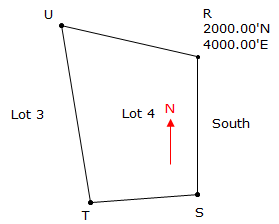Figure H-2 Lot 4 Survey

Both surveyors have good closure and compute coordinates for their lot corners.

Today, you are hired to do a survey in the vicinity of the two lots. Realizing that Jones' line CD and Davis' line TU are the same line, you decide to plot both their surveys in CAD. The result? Figure H-3.Figure H-3 Lots 3 and 4 Combined

Something doesn't look right - the two lots are supposed to be next to each other.

What happened?

The two surveys are in separate coordinate systems. Since each surveyor assumed his own starting coordinate and direction independent of each other, they have different coordinate origins and meridian direction. To resolve this problem we need to move Lot 3 into Lot 4's system or Lot 4 into Lot 3's system, Figure H-4 (we also have the option of moving both to a third system).Figure H-4 Correct Lots 3 and 4 Relationship

There are a few ways to do this, including a coordinate transformation. Regardless of the method, we must know something about how the systems are related to each other.

## 2. Coordinate Transformation

A coordinate transformation is used to change point positions from one coordinate system to another. Figure H-5 illustrates the same two sets of points from two different coordinate systems:Figure H-5 Two Points Known in Both Systems

The To system is final coordinate system. Points AT and BT are points correctly located in the To system. Points AF and BF are the same points but in a different coordinate system (From system). When plotted in the To system using their From coordinates they appear in a different location. Points CF and DF are also in the From system; we want their coordinates in the To system.

A transformation consists of three elements

• Rotation
• Scaling
• Translation

### a. Rotation

The From coordinates must be rotated to make directions coincide with those of the To system. To determine the rotation angle we need the direction of a common line in both systems.Figure H-6 Direction of Common Line in Both Systems

The difference in directions is the rotation angle, ρ, Figure H-7.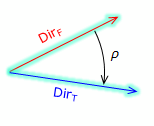Figure H-7 Rotation Angle

Rotation is computed from the direction difference of the same line in both systems. If using two control points, inversing between them in both systems will provide the requisite directions. From the directions the rotation is computed from: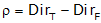Equation H-1

The rotation would be applied to the From coordinates creating a new set, subscripted 1 in Figure H-8:Figure H-8 Rotate From System

### b. Scaling

Scaling is used to increase or decrease distances between the points in order to make them fit in the new system. The distances may be off due to:

• random errors
• systematic errors
• ground to grid distortion (in larger regional systems)
• combinations of the above.

To scale the transformation, we need the length of the same line in both systems, Figure H-9. The length can be from direct measurement (and subsequent adjustment) or by inversing between the coordinates of two common points.Figure H-9 Length of Common Line in Both Systems

Scale is the ratio between the two lengths of the same line in both systems.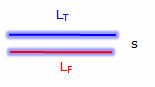Figure H-10 Scale

Scale is computed from:Equation H-2

Scaling isn't always necessary depending on the circumstances and allowable error. For example, if a maximum error of 1/5000 is acceptable, then a scale in the range of 1±1/5000 (0.9998 to 1.0002) can be ignored.

When accounting for scale there are three approaches:

1. Unitary - Maintains a 1:1 relationship between the two coordinate systems.

2. Uniform - Enforces a uniform scale (other than 1) in all directions. Only a single scale is computed for the entire transformation.

Both Unitary and Uniform approaches are referred to as a Conformal Transformation because true shapes are maintained.

3. Differential - Scale in the N/S (Y) direction differs from scale in the E/W (X) direction. Two different scales (SN and SE) are computed for the transformation. This is referred to as an Affine Transformation.

Once scale is applied another set of intermediate coordinates, subscripted 2, are created, Figure H-11:Figure H-11 Scaled From System

### c. Translation

The final element are two translations: shift positions in the N/S (Y) and E/W (X) directions. These are determined using coordinates from the previous step with the final coordinates of those points.Figure H-12 Common Point Displacement Between Systems

These two final elements complete the transformation: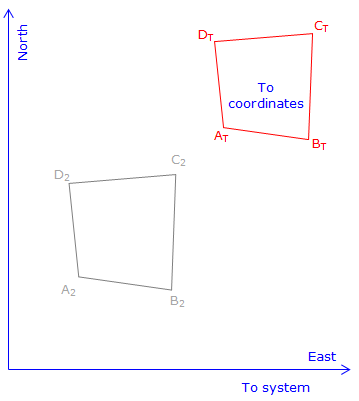Figure H-13 Translated From system

Translations are computed from a control point known in both systems (From: NF & EF; To: NT & ET) along with the rotation angle and scale previously determined.Equation H-3

A second control point, if available, can be used for a math check for the translation parameters.

### d. Number of parameters

A coordinate transformation is sometimes referred to as either a three-, four- or five-parameter transformation, Table H-1. The difference between the number of parameters is how scale is applied. Either way, a certain amount of data (control) is needed in both systems.

 Table H-1 Transformation type Three-parameter Four-parameter Five-parameter Parameters TN TE ρ TN TE ρ S TN TE ρ SN SE Control needed in both systems One point's coordinates, one line's directions One point's coordinates, one line's directions, one line's distances One point's coordinates, one line's directions two lines' distances or or or two points two points two points and one line's directions or three points

These are generally the minimal amounts of control needed to uniquely determine the transformation parameters. Any error in the control will be undiscovered and become part of the parameters affecting all points transformed.

Additional control data can be used for math checks. Due to the presence of random errors, different combinations of control will result in slightly different transformation parameters. To get a better model which propagates the error into the final positions, all control should be incorporated in a least squares transformation. Creating such a model manually is time consuming so we will not address least squares transformations here. Many surveying software packages usually include a least squares transformation as an option.

We will examine the four-parameter model as it works well for most ground-based survey applications. Including a uniform scale absorbs smaller random errors satisfactorily as long as good control is used.

### e. Transformation Equations

Once the parameters are determined, they are assembled to create the transformation equations which are used to move other points to the To system.Equation H-4 NT, ET are To system coordinates NF, EF are From system coordinates TE, TN are the translations in East and North directions ρ is the rotation angle S is the scale

## 3. Variations

While a full coordinate transformation includes rotation, scaling, and translation, there are situations where only one or two of the elements are necessary.

### a. Rotation only

An example where only a rotation is needed is converting magnetic to true directions. The traverse in Figure H-14 must be rotated through the declination angle to make the True North meridian coincide with the Magnetic North meridian.Figure H-14 Rotation Without Scale or Translation

A single traverse point can be used as a pivot; there is no scaling or translation.

### b. Translation only

When dealing with regional coordinate systems, the corners of a small survey may have large coordinates as shown in Figure H-15.Figure H-15 Large Coordinates in Formal System

To work with smaller coordinates, the surveyor may subtract one constant from all the North coordinates and another constant from the East coordinates. For traverse in Figure H-15 we could subtract 384,000.00 from the North coordinates and 2,307,000.00 from the East coordinates. The result would be Figure H-16.Figure H-16 Constants Subtracted From North and East Coordinates

This effectively creates a local origin:Figure H-17 Local Origin

Coordinate differences are still the same since each coordinate pair has been changed the same amount. Inverse and area computations are similarly unaffected outside the fact that the magnitude of the computations are somewhat simplified.

### c. Translation and rotation

A typical field situation could be collecting data referenced to a base line without first knowing the base line's location. The data is relative to the base line so later fixing the base line fixes the data.

A field crew sets up on one control station and uses another as a backsight for a topo survey. Not knowing coordinates of either point they assume the coordinates of A, their total station (TSI) location, and direction of the backsight line. From there they collect and reduce their topo data.

Later in the office, they are able to obtain point A's coordinates and the correct direction to point B, Figure H-18.Figure H-18 Data Collected Reference to Assumed Control

Using this information, they are able to translate and rotate the topo data to its correct location, Figure H-19.Figure H-19 Data Transformed Along with Control

## 4. Example

A crew surveyed the exterior of the parcel shown. They assumed the coordinates of the southernmost corner (1001) and used the record bearing for the southwesterly line (1001-1002).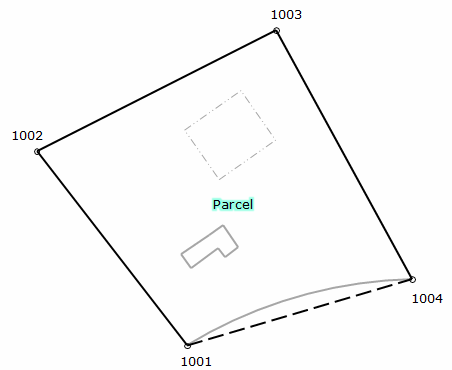Point North East 1001 3684.22 2208.05 1002 4030.04 1940.56 1003 4244.27 2366.04 1004 3802.06 2607.77
Figure H-20
Parcel Survey

Later, a second survey crew was sent out to locate the existing drainfield. They did not have the first crew's coordinates so they occupied the northernmost corner (NE), for which they assumed coordinates, and backsighted the westernmost corner (NW), for which they assumed a direction. They then shot the drainfield corners. As a check, they also shot the distance from NE to NW.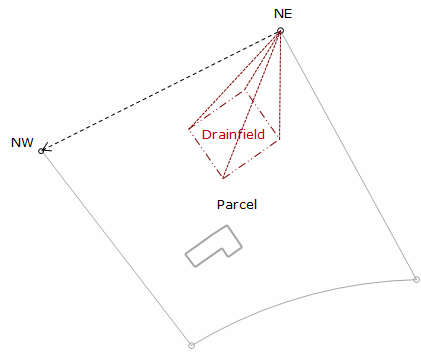Point North East NE 5000.00 3000.00 501 4914.03 2776.03 502 4814.98 2786.38 503 4829.01 2911.57 504 4934.06 2895.69
Figure H-21
Drainfield Survey

When the coordinates were brought together and plotted the results were as shwon in Figure H-22:Figure H-22 Parcel and Drainfield Combined

Using the boundary survey as the reference system, translate the drainfield data.

Determine transformation parameters

Step (1) Rotation angle

Line NE-NW coincides with line 1003-1002; line NE-NW must be rotated into line 1003-1002.Convert the bearings to azimuths and compute the rotation angle.

 Line NE-NWLine 1000-1002From Equation H-1:Step (2) Scale

Length of line NE-NW is 476.41', line 1003-1002 is 476.37'.

From Equation H-2:This represents a distortion of 1/(1-0.999916) = 1/11,900. If this is within tolerance, then the scale factor can be ignored. We will apply it in this example.

Step (3) Translations

Use the coordinates of points 1003 and NE in Equation H-3: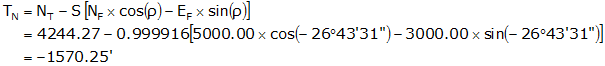Step (4) Math check

For a parameters math check, convert NW to see if it matches the coordinates of 1002.

In the From system, NW's coordinates are:Transform and check:Step (5) Transform points 501-504

Use Equation H-14 with the parameters computed in Steps (1)-(3)

Point 501Point 502Point 503Point 504## 5. Two-Dimensions vs Three-Dimensions

While the discussion here is limited to horizontal (two dimensional) coordinate systems, it is relatively easy to extend this into the third dimension by incorporating elevations or Z coordinates. An additional translation parameter would be needed for the elevation component, TElev.

The rotation angle, ρ, for a two dimensional transformation is a horizontal angle rotated about the vertical, or Z, axis. In a three dimensional transformation there would three rotation angles, one about each axis (X-Y-Z, or E-N-Elev).Figure H-23 Three Dimensional Transformation

Scale could again be Unitary, Uniform, or Differential. In the latter case, there would be three scale factors.

The total number of parameters in a three dimensional transformation could be:

• Six: Three translations, three rotations, 1:1 scale
• Seven: Three translations, three rotations, uniform scale
• Eight: Three translations, three rotations, one scale for horizontal, one scale for vertical
• Nine: Three translations, three rotations, three scales

The eight-parameter transformation would be used where there is a quality difference between horizontal and vertical positions in one system or the other.